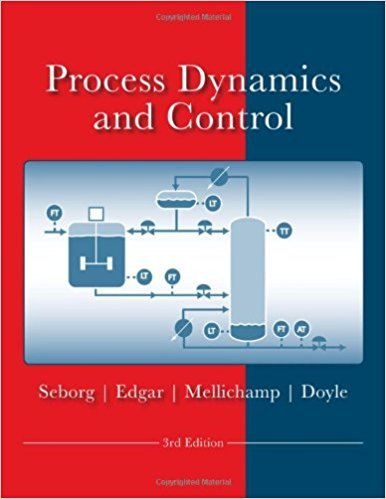×
Get Full Access to Process Dynamics And Control - 3 Edition - Chapter 1 - Problem 1.12
Get Full Access to Process Dynamics And Control - 3 Edition - Chapter 1 - Problem 1.12

×

# For the steam-heated tank shown below, identifyISBN: 9780470128671 148

## Solution for problem 1.12 Chapter 1

Process Dynamics and Control | 3rd Edition

• Textbook Solutions
• 2901 Step-by-step solutions solved by professors and subject experts
• Get 24/7 help from StudySoup virtual teaching assistantsProcess Dynamics and Control | 3rd Edition

4 5 1 431 Reviews
18
4
Problem 1.12

For the steam-heated tank shown below, identify manipulated, controlled, and disturbance variables. What disturbances are measured for feedforward control? How would the control system react to an increase in feed temperature in order to keep the tank temperature at its setpoint?

Step-by-Step Solution:
Step 1 of 3

Statistical Inference: provides methods for drawing conclusions about a population from the sample data. There are two ways to do this:  Confidence intervals: used to estimate the value of a population parameter using sample statistics Test of Significance: used for assessing evidence for a claim about the  population To set up  Have to have an SRS from the population, no bias  The variable has to have an exactly normal distribution in the population  We don’t know population mean , but we know the population standard deviation Confidence Interval  What statistic can be used to estimate the population parameter o We can use x-bar  To do a confidence interval, it is similar to the 68-95-99.7 rule. So you can say with 95% confidence, the mean number or something will fall between two standard deviations of the mean. o The sampling distribution tells us how close to the mean that the sample mean is o A statistical Estimation: tells us how close to the sample mean x bar the unknown mean is likely to be o Margin of Error: shows how accurate we believe out guess is on the variability of our estimate  The confidence interval should be in the format: estimate +- margin of error  Formula: margin of error = z*(standard deviation/sqrt(n)) o Confidence level  An interval calculated from the sample data: x bar +_ the margin of error  A confidence level C: the success rate of the method that produces the interval, which gives the probability that the interval will capture the true parameter value with repeated sampling o Format: "We are C% confident that our interval captures the unknown mean"  Critical z values o Represented by z*  Common z* values o Confidence 90% 95% 99% Level C Critical value z* 1.645 1.960 2.57 6 Steps for making confidence intervals 1 Identify the parameter and pick a level of confidence. 2 You have to check the conditions first to use the interval, then calculate it 3 Interpret the results in a format of " I am __% confident that the mean of ___ lies between ___ and ___." How to find confidence interval (assuming requirements for a confidence interval are fulfilled: 1 Estimate the mean and standard deviation for the population. The mean is the same as the sample mean, and the standard deviation is 1 Find the critical value: If the question is asking for a 95% confidence interval, then do Norm.s.inv(.95), and the positive and negative of this are your intervals 2 Calculate the margin of error using the formula: 1 The mean +- the margin of error is your final interval 2 "I am 95% confident that the true mean of ___ lies between __ and __" Tests of Significance  Tests of significance are used to prove or disprove a certain claim, such as if a person were to say that they make 90% of their basketball shots, and in a test they only made 3 out of 10. you could use a test of significance to prove that they were lying.  Parts of a significance test 1. State your hypothesis  There are two hypotheses, The null hypothesis: H andothe alternative hypothesis: H The null hypothesis is always the a. claim you are testing originally. The alternative hypothesis is going to be what you think will actually happen, or what is going against the null hypothesis.  The alternative hypothesis can be one sided( if it states the parameter is larger or smaller than H )oor two sided (if it states that the parameter is ≠ to the H o 2. Calculate the test statistics 3. Calculate the p value 4. Make a conclusion

Step 2 of 3

Step 3 of 3

##### ISBN: 9780470128671

Since the solution to 1.12 from 1 chapter was answered, more than 578 students have viewed the full step-by-step answer. This full solution covers the following key subjects: control, temperature, tank, Keep, disturbances. This expansive textbook survival guide covers 24 chapters, and 420 solutions. The full step-by-step solution to problem: 1.12 from chapter: 1 was answered by , our top Engineering and Tech solution expert on 11/15/17, 04:03PM. This textbook survival guide was created for the textbook: Process Dynamics and Control, edition: 3. Process Dynamics and Control was written by and is associated to the ISBN: 9780470128671. The answer to “For the steam-heated tank shown below, identify manipulated, controlled, and disturbance variables. What disturbances are measured for feedforward control? How would the control system react to an increase in feed temperature in order to keep the tank temperature at its setpoint?” is broken down into a number of easy to follow steps, and 41 words.

Unlock Textbook Solution